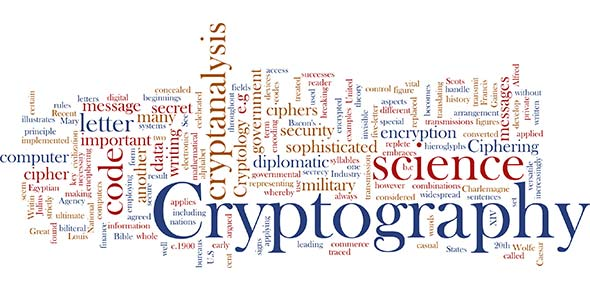# CISSP-cryptography

17 Questions | Total Attempts: 7782Settings• 1.
Asymmetric key cryptography is used for all of the following except:
• A.

Encryption of data

• B.

Access control

• C.

Nonrepudiation

• D.

Steganography

• 2.
The most common forms of asymmetric key cryptography include
• A.

Diffie–Hellman

• B.

Rijndael

• C.

Blowfish

• D.

SHA-256

• 3.
What is an important disadvantage of using a public key algorithm compared to a symmetric algorithm?
• A.

A symmetric algorithm provides better access control.

• B.

A symmetric algorithm is a faster process.

• C.

A symmetric algorithm provides nonrepudiation of delivery.

• D.

A symmetric algorithm is more diffi cult to implement.

• 4.
When a user needs to provide message integrity, what options may be best?
• A.

Send a digital signature of the message to the recipient

• B.

Encrypt the message with a symmetric algorithm and send it

• C.

Encrypt the message with a private key so the recipient can decrypt with the corresponding public key

• D.

Create a checksum, append it to the message, encrypt the message, then send to recipient.

• 5.
A certifi cate authority provides what benefi ts to a user?
• A.

Protection of public keys of all users

• B.

History of symmetric keys

• C.

Proof of nonrepudiation of origin

• D.

Validation that a public key is associated with a particular user

• 6.
What is the output length of a RIPEMD-160 hash?
• A.

160 bits

• B.

150 bits

• C.

128 bits

• D.

104 bits

• 7.
ANSI X9.17 is concerned primarily with
• A.

Protection and secrecy of keys

• B.

Financial records and retention of encrypted data

• C.

Formalizing a key hierarchy

• D.

The lifespan of key-encrypting keys (KKMs)

• 8.
When a certificate is revoked, what is the proper procedure?
• A.

Setting new key expiry dates

• B.

Updating the certificate revocation list

• C.

Removal of the private key from all directories

• D.

Notification to all employees of revoked keys

• 9.
• A.

• B.

• C.

Link encryption is suitable for high-risk environments.

• D.

Link encryption provides better traffi c fl ow confi dentiality.

• 10.
A_________ is the sequence that controls the operation of the cryptographic algorithm.
• A.

Encoder

• B.

Decoder wheel

• C.

Cryptovariable

• D.

Cryptographic routine

• 11.
The process used in most block ciphers to increase their strength is
• A.

Diffusion

• B.

Confusion

• C.

Step function

• D.

SP-network

• 12.
The two methods of encrypting data are
• A.

Substitution and transposition

• B.

Block and stream

• C.

Symmetric and asymmetric

• D.

DES and AES

• 13.
Cryptography supports all of the core principles of information security except
• A.

Availability

• B.

Confidentiality

• C.

Integrity

• D.

Authenticity

• 14.
A way to defeat frequency analysis as a method to determine the key is to use
• A.

Substitution ciphers

• B.

Transposition ciphers

• C.

Polyalphabetic ciphers

• D.

Inversion ciphers

• 15.
Th e running key cipher is based on
• A.

Modular arithmetic

• B.

XOR mathematics

• C.

Factoring

• D.

Exponentiation

• 16.
The only cipher system said to be unbreakable by brute force is
• A.

AES

• B.

DES

• C.

• D.

Triple DES

• 17.
Messages protected by steganography can be transmitted to
• A.

Picture files

• B.

Music files

• C.

Video files

• D.

All of the above

Related TopicsBack to top